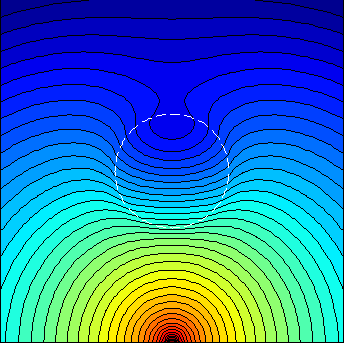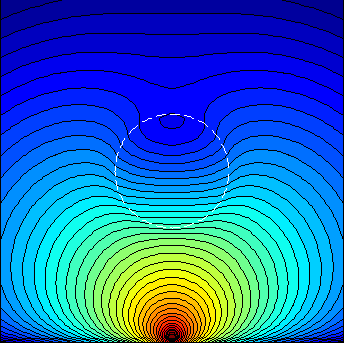# Matlab Toolbox for the Analytical Diffusion/Helmholtz Solutions of a Sphere

The "Sphere-Diffusion" toolbox solves for the analytical solutions for the diffusion inside and outside a sphere. This toolbox is part of an open-source software, MMC, developed by Qianqian Fang. The license of the toolbox is GNU General Public License version 3. Please see LICENSE.txt for details.

The solutions are defined in the 3D space on a user specified grid; the sphere can have different absorption/scattering/refractive index to the background media. This toolbox can be useful when evaluating new algorithms in the heterogeneous media. The toolbox is compatible with GNU Octave.

2. Core functions
3. Contour plots of the sample solutions
3.1.1. A 10mm sphere inside an infinite homogeneous medium
3.1.2. A 10mm sphere inside a semi-infinite homogeneous medium
3.1.3. A 10mm sphere inside an infinite homogeneous slab
4. Other functions
6. Examples
7. Acknowledgement
8. Reference

The author of the toolbox is appreciated if you can cite the references, [Fang2010] and [Boas1994], listed at the end of this document if you choose to use this toolbox in your publication.

## 2. Core functions

[res,xi,yi,zi] = sphdiffusioninfinite(xrange,yrange,zrange,cfg)
diffusion solution for a sphere inside the infinite homogeneous medium
[res,xi,yi,zi] = sphdiffusionsemi(Reff,xrange,yrange,zrange,cfg)
diffusion solution for a sphere inside a semi-infinite homogeneous medium
[res,xi,yi,zi] = sphdiffusionslab(Reff1,Reff2,h,xrange,yrange,zrange,cfg)
diffusion solution for a sphere inside an infinite homogeneous slab

## 3. Contour plots of the sample solutions

#### 3.1.1. A 10mm sphere inside an infinite homogeneous medium#### 3.1.2. A 10mm sphere inside a semi-infinite homogeneous medium#### 3.1.3. A 10mm sphere inside an infinite homogeneous slab## 4. Other functions

function description
besselhprime.m Hankel function first order derivative
besseljprime.m Bessel function (Bessel first kind) first order derivative
besselyprime.m Neumann function (Bessel second kind) first order derivative
spbesselh.m Spherical Hankel function
spbesselhprime.m Spherical Hankel function first order derivative
spbesselj.mSpherical Bessel function
spbesseljprime.m Spherical Bessel function first order derivative
spbessely.mSpherical Neumann function
spbesselyprime.m Spherical Neumann function first order derivative
spharmonic.mSpherical harmonics
sphdiffAcoeff.mSphere exterior field coefficients
sphdiffBcoeff.mSphere exterior field coefficients
sphdiffCcoeff.mSphere interior field coefficient
sphdiffexterior.m Sphere exterior total field
sphdiffincident.m Incident field
sphdiffinterior.m Sphere interior field
sphdiffscatter.mSphere exterior scattered field

## 5. Comments on semi-infinite solutions

see the comments in the sphdiffusionsemi.m script

``` % high order terms are ignored, for example, the Phi(S2,O2) scattered by
% real sphere O1, or Phi(S1,O1) scatted by the mirrored sphere O2 etc
%
% 1st order approximation works only when the sphere is far away from
% source and interface so that multiple-scattering is weak.
%
%                      ____
%                    .'    `. real sphere
%                   /   O1   \
%                   |    o----|-----> x
%                   \    | a /  phi_interior=phi_interior(S1,O1)
%                    `.__|_.'               +phi_interior(S2,O1)
%                        |  phi_ext=phi_incident(S1,O1)+phi_scatter(S1,O1)
%                        |     - phi_incident(S2,O2)-phi_scatter(S2,O2)
%                        |     + phi_scatter(S1,O2) -phi_scatter(S2,O2)
%                        |     + high order terms(phi_scatter(S1,O1,O2),..)
%                     S1 x  real source
% _______________________|____________________z0______ true boundary
%                        |                    zb
% -----------------------|---------------------- extrapolated boundary
%           ^            |                    zb
%           |            |                    z0
%           |         S2 x  mirrored source  ---  S2=-S1
%           |            |
%           | src0(1)    |
%           |            |
%           |          ..|.  mirrored sphere
%           |        .'  | `.
%           v       /    | a \  (origin for the mirrored field)
%           ------- :    o----:-----> x
%                   \   O2   /
%                    `......'
%
%
```

## 6. Examples

``` %%%%%%%%%%%%%%%%%%%%%%%%%%%%%%%%%%%%%%%%%%%%%%%%
% setting up problem domain
%%%%%%%%%%%%%%%%%%%%%%%%%%%%%%%%%%%%%%%%%%%%%%%%
cfg.v=299792458000;  % speed-of-light in mm/s
cfg.a=10;            % radius of the sphere, mm
cfg.omua=0.002;      % outside (background) mua 1/mm
cfg.omusp=0.990;     % outside (background) mus' 1/mm
cfg.imua=0.050;      % inside (sphere) mua 1/mm
cfg.imusp=0.500;     % inside (sphere) mus' 1/mm
%cfg.imua=0.002;
%cfg.imusp=0.990;
cfg.src=[30,pi,0];   % source position in spherical coordinates
cfg.maxl=20;         % maximum orders for the series expansion
cfg.omega=0;         % modulation frequency
```

``` cfg.Din=cfg.v/(3*cfg.imusp);  % Diffusion coefficient in the sphere
cfg.Dout=cfg.v/(3*cfg.omusp); % Diffusion coefficient outside the sphere
cfg.kin=sqrt((-cfg.v*cfg.imua+i*cfg.omega)/cfg.Din);   % complex-wavenumber in the sphere
cfg.kout=sqrt((-cfg.v*cfg.omua+i*cfg.omega)/cfg.Dout); % complex-wavenumber outside the sphere
```

``` % solution of the sphere in an infinite medium
[phi_ana,xa,ya,za]=sphdiffusioninfinite(-30:0.8:30,0,-30:0.8:30,cfg);
figure;contourf(xa,za,log10(abs(phi_ana)),40);axis equal;
```

``` % solution of the sphere in an infinite slab with a height of 60mm
[phi_ana,xa,ya,za]=sphdiffusionslab(0,0,60,-30:0.8:30,0,-30:0.8:30,cfg);
figure;contourf(xa,za,log10(abs(phi_ana)),40);axis equal;
```

## 7. Acknowledgement

Qianqian Fang would like to thank David Boas for the helpful discussions on the semi-infinite solutions.

## 8. Reference

• Q. Fang, "Mesh-based Monte Carlo method using fast ray-tracing in Plücker coordinates," Biomed. Opt. Express 1, 165-175 (2010)
• D. A. Boas, M. A. O’Leary, B. Chance, and A. G. Yodh, “Scattering of diffuse photon density waves by spherical inhomogeneities within turbid media: analytic solution and applications,” Proc. Natl. Acad. Sci. U.S.A. 91(11), 4887–4891 (1994).